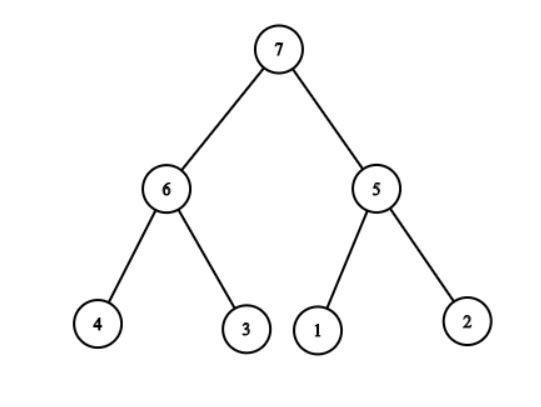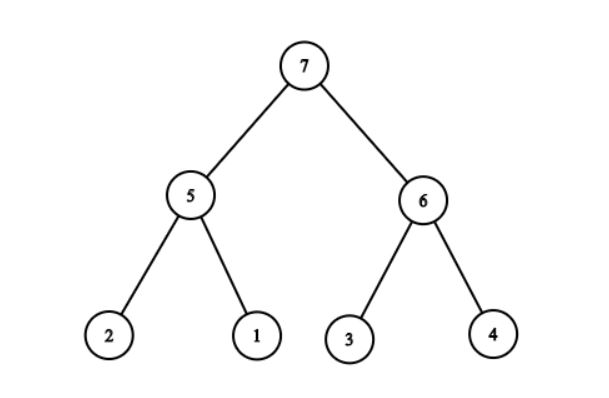Implementation and Conversion Based Problems
0% completed
Build Heap
Convert Min-Heap to Max-Heap
Convert BST to Min Heap2

# Convert Min-Heap to Max-Heap

Difficulty: MEDIUM
Avg. time to solve
26 min

Problem Statement
Suggest Edit

#### You are given an array/list (arr) representing a min-heap. Your task is to write a function that converts the min-heap to a max-heap.

##### Note :
``````Change in the input array/list itself. You don't need to return or print the elements.
``````
##### Input format :
``````The first line contains an integer 't' which denotes the number of test cases or queries to be run. Then the test cases follow.

The first line of each test case or query contains an integer 'N' representing the size of the array/list.

The second line contains 'N' single space separated integers representing the elements in the array/list which are in the form of a min-heap.
``````
##### Output Format :
``````For each test case or query, If the built max-heap satisfies the max-heap condition, print 'true'. Otherwise, print 'false'(without quotes).

Output for every test case will be printed in a separate line.
``````
##### Note :
``````You don't have to print anything, it has already been taken care. Build the max-heap in the given array/list itself or in-place
``````
##### Constraints :
``````1 <= t <= 100
0 <= N <= 10^5

Time Limit: 1 sec
``````
##### Sample Input 1 :
``````1
7
1 2 3 4 5 6 7
``````
##### Sample Output 1 :
``````true
``````
##### Explanation for Input 1:
``````For a min-heap, there can be multiple max heap. For example:
``````Since it is possible to create a max heap so answer should be true.

##### Sample Input 2 :
``````1
2
1 2
``````
##### Sample Output 2 :
``````true
``````
##### Explanation for Input 2 :
``````In this case, there is only one max heap possible.
``````Console